Courses

# Test: What Is Long, What Is Round?

## 10 Questions MCQ Test Mathematics (Maths) for Class 2 | Test: What Is Long, What Is Round?

Description
This mock test of Test: What Is Long, What Is Round? for Class 2 helps you for every Class 2 entrance exam. This contains 10 Multiple Choice Questions for Class 2 Test: What Is Long, What Is Round? (mcq) to study with solutions a complete question bank. The solved questions answers in this Test: What Is Long, What Is Round? quiz give you a good mix of easy questions and tough questions. Class 2 students definitely take this Test: What Is Long, What Is Round? exercise for a better result in the exam. You can find other Test: What Is Long, What Is Round? extra questions, long questions & short questions for Class 2 on EduRev as well by searching above.
QUESTION: 1

### A square could also be called a...Solution:

Rectangles must have 4 sides, four 90 degree angles and two pairs of parallel sides

QUESTION: 2

### This pattern is made up of...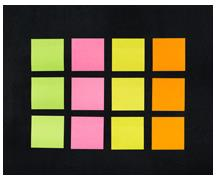Solution:

There are rows of squares in this pattern

QUESTION: 3

### This is a pattern made of..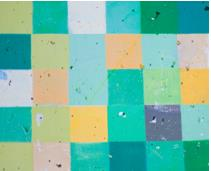Solution:

There are rows and rows of squares in the pattern

QUESTION: 4

What shape is this person making with their hands?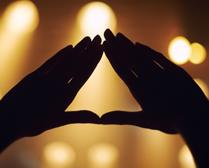Solution:

They are making the three sides of a triangle

QUESTION: 5

What shape is the clock?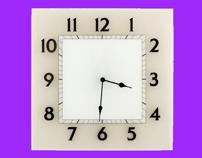Solution:

It has four equal sides

QUESTION: 6

These people are standing in a...Solution:

They are making a round shape - a circle

QUESTION: 7

These bricks are all..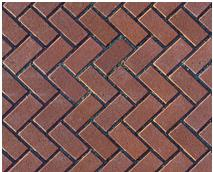Solution:

The oblongs are making a herringbone pattern

QUESTION: 8

What is the shape of frame around these snooker balls?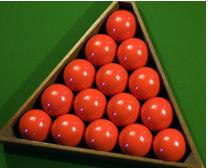Solution:

It's a triangular frame

QUESTION: 9

What shape makes up this pattern?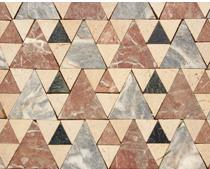Solution:

They are large and small triangles

QUESTION: 10

The board is a...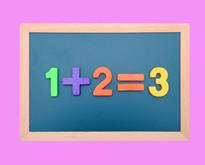Solution:

The board is rectangular and also an oblong